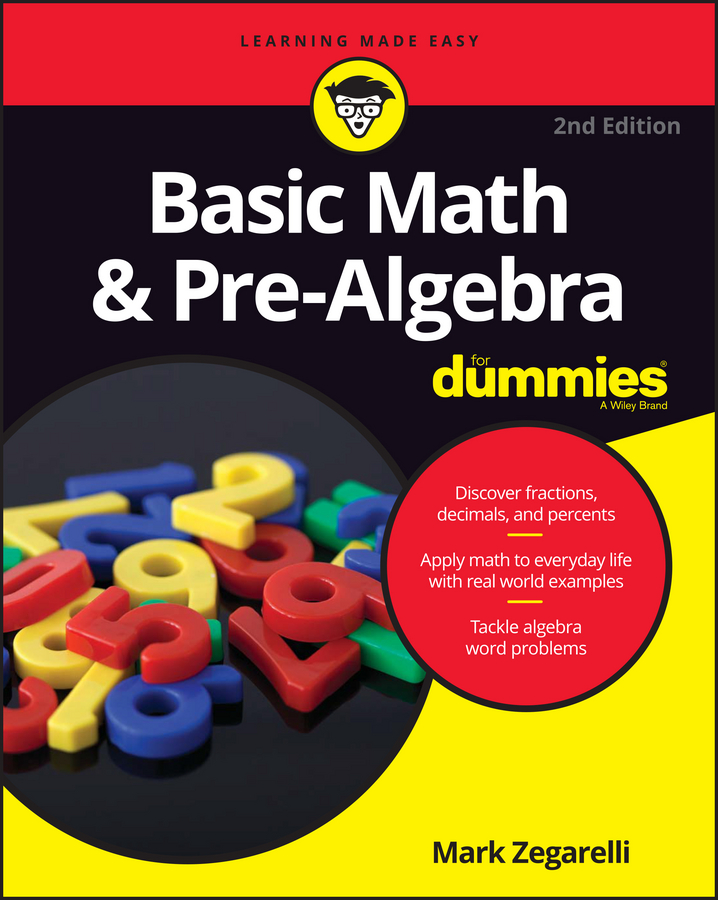##### Basic Math & Pre-Algebra For DummiesSubtraction is usually the second operation you discover, and it’s not much harder than addition. In fact, you can subtract larger numbers by stacking them in columns, similar to how you add large numbers.

Still, there’s something negative about subtraction — it’s all about who has more and who has less. Suppose you and your friend have been running on treadmills at the gym. You’re happy because you ran 3 miles, but then she starts bragging that she ran 10 miles. She subtracts and tells you that you should be very impressed that she ran 7 miles farther than you did. (But with an attitude like that, she shouldn’t be surprised if she comes back from the showers to find her running shoes filled with liquid soap!)

As with addition, subtraction has only one sign: the minus sign (–). You end up with equations such as 4 – 1 = 3 and 14 – 13 = 1 and 93 – 74 = 19.

When you subtract one number from another, the result is called the difference. This term makes sense when you think about it: When you subtract, you find out the difference between a higher number and a lower one.

In subtraction, the first number is called the minuend, and the second number is called the subtrahend. But almost nobody ever remembers which is which, so when talking about subtraction, you may prefer to say the first number and the second number.

One of the first things you probably heard about subtraction is that you can’t take away more than you start with. In that case, the second number can’t be larger than the first. And if the two numbers are the same, the result is always 0. For example, 3 – 3 = 0 and 11 – 11 = 0 and 1,776 – 1,776 = 0. Later, someone breaks the news that you can take away more than you have. When you do, though, you need to place a minus sign in front of the difference to show that you have a negative number, a number below 0:

4 – 5 = –1

10 – 13 = –3

88 – 99 = –11

When subtracting a larger number from a smaller number, remember the words switch and negate. That is, switch the order of the two numbers and do the subtraction as you normally would, but at the end negate the result by attaching a minus sign. For example, to find 10 – 13, you switch the order of these two numbers, giving you 13 – 10, which equals 3; then negate this result to get –3. That’s why 10 – 13 = –3.

The minus sign gets double duty, so don’t get confused. When you stick a minus sign between two numbers, it means the first number minus the second number. But when you attach it to the front of a number, it means that this number is a negative number.

To subtract larger numbers, stack one on top of the other as you do with addition. (For subtraction, however, don’t stack more than two numbers — put the larger number on top and the smaller under it.) For example, suppose you want to subtract 386 – 54. To start out, stack the two numbers and begin subtracting in the ones column: 6 – 4 = 2:

386

– 54

2

Next, move to the tens column and subtract 8 – 5 to get 3:

386

54

32

Finally, move to the hundreds column. This time, 3 – 0 = 3:

386

– 54

332

This problem shows you that 386 – 54 = 332.